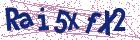# Fitted scheme for singularly perturbed time delay reaction-diffusion problems

Document Type : Research Article

Authors

Department of Mathematics, College of Sciences, Bahir Dar University, Bahir Dar, Ethiopia.

Abstract

In this article, we constructed a numerical scheme for singularly perturbed time-delay reaction-diffusion problems. For the discretization of the time derivative, we used the Crank-Nicolson method and a hybrid scheme, which is a combination of the fourth-order compact difference scheme and the cen-tral difference scheme on a special type of Shishkin mesh in the spatial di-rection. The proposed scheme is shown to be second-order accurate in time and fourth-order accurate with a logarithmic factor in space. The uniform convergence of the proposed scheme is discussed. Numerical investigations are carried out to demonstrate the eﬀicacy and uniform convergence of the proposed scheme, and the obtained numerical results reveal the better per-formance of the present scheme, as compared with some existing methods in the literature.

Keywords20.1001.1.24236977.2023.13.2.7.0

Main Subjects

#### References

 Ansari, A., Bakr, S. and Shishkin, G. A parameter-robust finite differ-ence method for singularly perturbed delay parabolic partial differential equations, J. Comput. Appl. Math. 205 (2007), 552–566.
 Babu, G. and Bansal, K. A high order robust numerical scheme for sin-gularly perturbed delay parabolic convection diffusion problems, J. Appl. Math. Comput. 68 (2021), 363–389.
 Clavero, C. and Gracia, J.L. High order methods for elliptic and time dependent reaction–diffusion singularly perturbed problems, Appl. Math. Comput. 168 (2005), 1109–1127.
 Clavero, C. and Gracia, J.L. On the uniform convergence of a finite dif-ference scheme for time dependent singularly perturbed reaction-diffusion problems, Appl. Math. Comput. 216 (2010), 1478–1488.
 Clavero, C. and Gracia, J.L. A high order hodie finite difference scheme for 1d parabolic singularly perturbed reaction–diffusion problems, Appl. Math. Comput. 218 (2012), 5067–5080.
 Clavero, C., Gracia, J. and Jorge, J. High-order numerical methods for one-dimensional parabolic singularly perturbed problems with regular lay-ers, Numer. Methods Partial Differential Equations 21 (2005), 149–169.
 Gelu, F.W. and Duressa, G.F. A uniformly convergent collocation method for singularly perturbed delay parabolic reaction-diffusion prob-lem, Abstr. Appl. Anal. 2021 (2021).
 Govindarao, L. and Mohapatra, J. A second order numerical method for singularly perturbed delay parabolic partial differential equation, Eng. Comput.
 Govindarao, L., Mohapatra, J. and Das, A. A fourth-order numerical scheme for singularly perturbed delay parabolic problem arising in popu-lation dynamics, J. Appl. Math. Comput. 63 (2020), 171–195.
 Govindarao, L. and Mohapatra, J. Numerical analysis and simulation of delay parabolic partial differential equation involving a small parameter, Eng Comput 37 (2019), 289–312.
 Kadalbajoo, M.K. and Awasthi, A. Crank–nicolson finite difference method based on a midpoint upwind scheme on a non-uniform mesh for time-dependent singularly perturbed convection–diffusion equations, Int. J. Comput. Math. 85 (2008), 771–790.
 Kumar, P.M.M. and Kanth, A.R. Computational study for a class of time-dependent singularly perturbed parabolic partial differential equation through tension spline, Comput. Appl. Math. 39 (2020), 1–19.
 Kumar, S. and Kumar, M. High order parameter-uniform discretization for singularly perturbed parabolic partial differential equations with time delay, Comput. Math. Appl. 68 (2014), 1355–1367.
 Kumar, M. and Rao, S.C.S. High order parameter-robust numerical methodfor time dependent singularly perturbed reaction–diffusion prob-lems, Computing 90 (2010), 15–38.
 Longtin, A. and Milton, J.G. Complex oscillations in the human pupil light reflex with mixed and delayed feedback, Math. Biosci. 90 (1988), 183–199.
 Mackey, M.C. and Glass, L. Oscillation and chaos in physiological control systems, Science 197 (1977), 287–289.
 Mallet-Paret, J. and Nussbaum, R.D. A differential-delay equation aris-ing in optics and physiology, SIAM J. Math. Anal. 20 (1989), 249–292.
 Miller, J. ORiordan, E. and Shishkin, G. Fitted numerical methods for singular perturbation problems, rev. edn, 2012.
 Mohapatra, J. and Govindarao, L. A fourth order optimal numerical approximation and its convergence for singularly perturbed time delayed parabolic problems, Iranian Journal of Numerical Analysis and Optimiza-tion (2021).
 Negero, N.T. and Duressa, G.F. Uniform convergent solution of singu-larly perturbed parabolic differential equations with general temporal-lag, Iran. J. Sci. Technol. Trans. A Sci. 46 (2022), 507–524.
 Priyadarshana, S., Mohapatra, J. and Govindrao, L. An eﬀicient uni-formly convergent numerical scheme for singularly perturbed semilinear parabolic problems with large delay in time, J. Appl. Math. Comput. 68 (2021), 2617–2639.
 Priyadarshana, S., Mohapatra, J. and Pattanaik, S.R.Parameter uni-form optimal order numerical approximations for time-delayed parabolic convection diffusion problems involving two small parameters, J. Com-put. Appl. Math. 41 (2022).
 Sahu, S.R. and Mohapatra, J. Numerical investigation of time delay parabolic differential equation involving two small parameters, Eng. Com-put. 38 (2021), 2882–2899.
 Salama, A. and Al-Amery, D. Asymptotic-numerical method for singu-larly perturbed differential difference equations of mixed-type, J. Appl. Math. Inform. 33 (2015), 485–502.
 Salama, A. and Al-Amery, D. A higher order uniformly convergent method for singularly perturbed delay parabolic partial differential equa-tions, Int. J. Comput. Math. 94 (2017), 2520–2546.
 Vulanović, R. A higher-order scheme for quasilinear boundary value prob-lems with two small parameters, Computing 67 (2001), 287–303.
 Wazewska-Czyzewska, M. and Lasota, A. Mathematical models of the red cell system, Matematyta Stosowana 6 (1976), 25–40.
 Yadav, S. and Rai, P. A higher order scheme for singularly perturbed delay parabolic turning point problem, Eng. Comput. 38 (2020), 819–851.